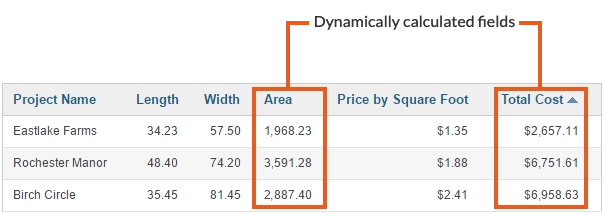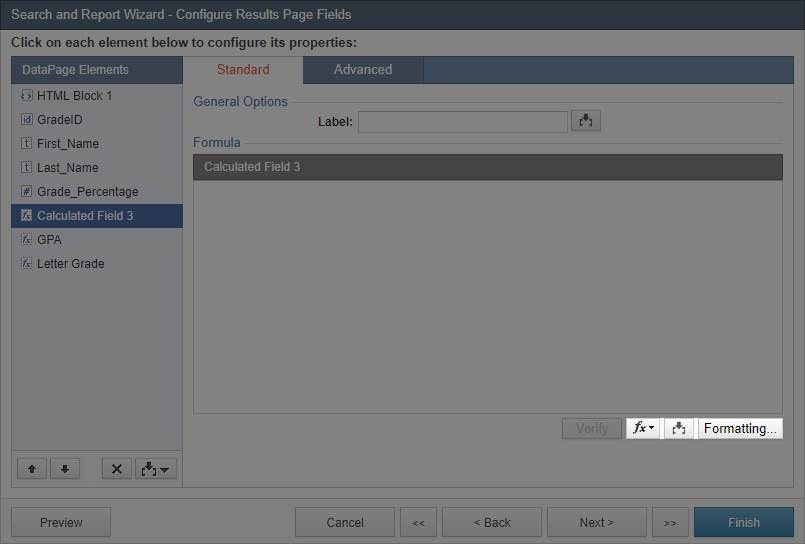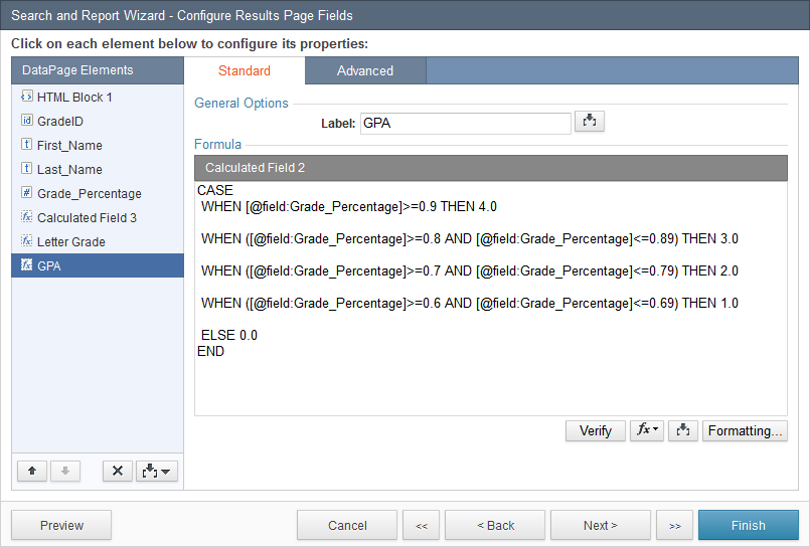Calculated fields allow you to perform dynamic, per-record functions on results and details pages of a Report DataPage. The value in a Calculated field is determined dynamically when the DataPage loads and does not affect the data in your table. Many functions are provided for data calculations and analysis including mathematical, statistical, logical, and string functions. Calculated fields can also receive and process passed parameters for further flexibility.Calculated fields primarily (but not always) operate on the values from a single record. For example, if your table has a length and a width field, you can use a Calculated field to determine and display the “area” for each record by multiplying width by length.

The following related features are described separately:

Note: Calculated field is a Formulas and Calculations feature that is not available in some plans.

To add a Calculated field, click the Insertbutton at the lower left of the Configure Results Page Fields screen in your DataPage wizard.Note: Caspio allows up to 50 Calculated fields in Results/Details Pages.

When creating a Calculated field, you can choose a label and create a formula to generate your Calculated field data. At the bottom right of the Calculated field’s Formula panel there are three buttons to help you create a formula.To view and select a function, click the Functionbutton.
You can use the Insertbutton to add fields and parameters to use with formulas. These parameters will be dynamically replaced by actual data from the record at run time.

To include Unicode characters such as extended Latin characters, symbols, emoji or non-Latin characters in the formula, add the N prefix to the string, for example: N’Ausländer’. Some Unicode characters are ignored in comparisons.

Finally, the Formatting button allows you to choose the display format of the calculated value. You can assign a formatting from the localization of the DataPage, or specify a custom formatting.

### Calculated Field Examples

Example 1: Calculates the Total Cost by multiplying Area by Price per Square Foot and rounds it to two decimal places.

`Round([@field:Area]*[@field:Price_per_Square_Foot],2)`

Example 2: Calculates Sales for the first half of the year and rounds it to a whole number.

`Round([@field:Q1_Sales]+[@field:Q2_Sales],0)`

Example 3: Determines Age by calculating the years between Today and field containing Date of Birth. Learn more.

### Conditional Cases in Calculated Fields

You can use the CASE expression to display data based on conditions.

Example 4: The example below illustrates how the CASE expression may be used. The Grade field is used to determine and display PASSED or FAILED, a letter grade and the GPA for each student.In the first Calculated Field, we have specified that if the Grade is greater than or equal to 60%, the value is PASSED, otherwise FAILED is returned.Note: Use single quotes around strings of text.
In the next Calculated Field, the Grade is compared to a range of values to determine a letter grade for each student.Similarly, the GPA is determined by comparing the Grade to a range of values.Example 5: In this example, the condition is applied to a text field. Depending on the value of Payment_Status, a string of text is provided.
```CASE
WHEN [@field:Payment_Status]='Overdue' THEN 'Contact: [@field:First_Name] [@field:Last_Name] ASAP'

ELSE 'No action needed'
END
```
Note: The IF/THEN/ELSE expression can’t be used in Calculated fields. However, you can achieve the same by using a CASE expression.

### Subqueries in Calculated Fields

Calculated fields also support the SELECT statement to get values from the same or other tables.
Note: If you want to select data from a View, add prefix _v_ to the View name.
Example 6: On the Report DataPage which shows conversation details from the tbl_Conversation table we will show the Description field from the tbl_Customers table. To do so:
1. On the report for which the data source is the tbl_Conversation table, add a Calculated field.
2. In the Formula field write:
`SELECT Description FROM tbl_Customers WHERE CustomerID=target.[@field:CustomerID]`
• This statement returns the Description field from tbl_Customers table whose Customer_ID is equal to target.[@field:Customer_ID].
• The target.[@field:Customer_ID] refers to the Customer_ID of the current row in the report/Details DataPage.Example 7: On the Report DataPage which shows customer details from the tbl_Customers table we will show the number of conversations based on the information in the tbl_Conversations table.
`SELECT Count(ConversationID) FROM tbl_Conversations WHERE CustomerID=target.[@field:CustomerID]`